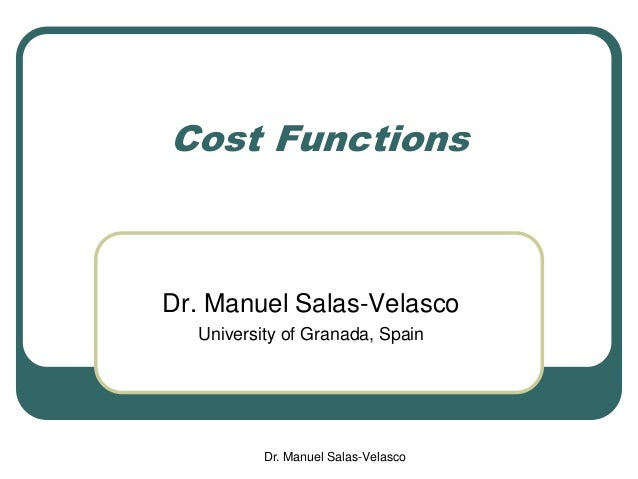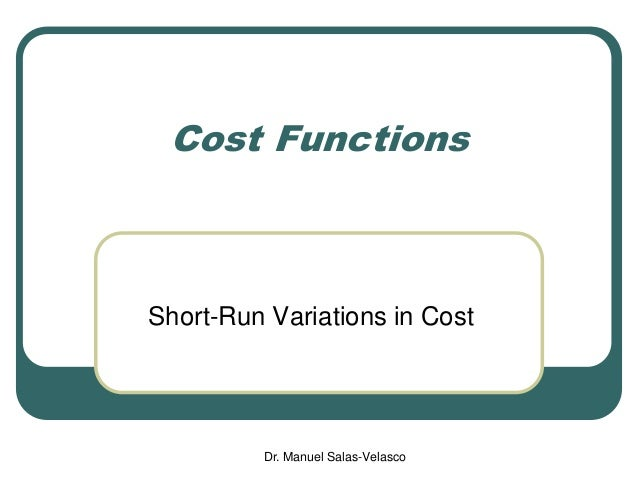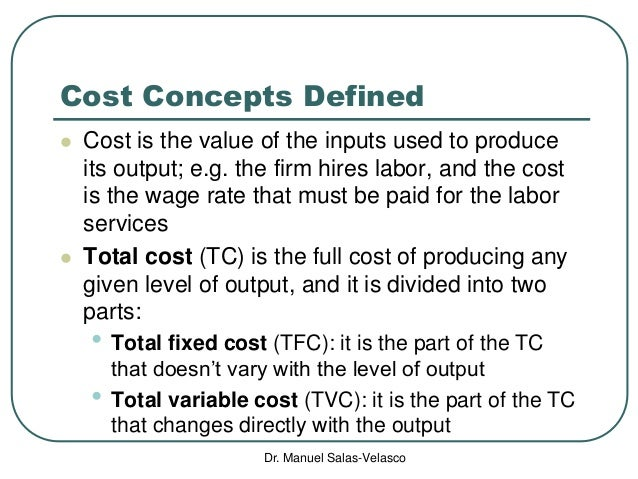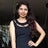Successfully reported this slideshow.Upcoming SlideShare
×

ofUpcoming SlideShare
Microeconomics: Production Theory
Next

182

Share

# Microeconomics: Cost Functions

Cost Functions
Cost Concepts Defined
Short-Run Cost Curves
Long-Run: Optimal Combination of Inputs
Constrained Cost Minimization: Lagrangian Multiplier Method
The U Shape of the LAC Curve

See all

See all

### Microeconomics: Cost Functions

1. 1. Cost Functions Dr. Manuel Salas-Velasco University of Granada, Spain Dr. Manuel Salas-Velasco
2. 2. Dr. Manuel Salas-Velasco Cost Functions Short-Run Variations in Cost
3. 3. Dr. Manuel Salas-Velasco Cost Concepts Defined  Cost is the value of the inputs used to produce its output; e.g. the firm hires labor, and the cost is the wage rate that must be paid for the labor services  Total cost (TC) is the full cost of producing any given level of output, and it is divided into two parts: • Total fixed cost (TFC): it is the part of the TC that doesn’t vary with the level of output • Total variable cost (TVC): it is the part of the TC that changes directly with the output
4. 4. Dr. Manuel Salas-Velasco Average Costs  Average total cost (ATC) is the total cost per unit of output outputofunits TC ATC   Average fixed cost (AFC) is the fixed cost per unit of output  Average variable cost (AVC) is the variable cost per unit of output outputofunits TFC AFC  outputofunits TVC AVC 
5. 5. Dr. Manuel Salas-Velasco Marginal costs  Marginal cost (incremental cost) is the increase in total cost resulting from increasing the level of output by one unit  Since some of total costs are fixed costs, which do not change as the level of output changes, marginal cost is also equal to the increase in variable cost, that results when output is increased by one unit Q TC MC    outputinChange costtotalinChange
6. 6. Dr. Manuel Salas-Velasco Cost Functions Short-Run Cost Curves
7. 7. Dr. Manuel Salas-Velasco Short Run versus Long Run  Short run • At least one input is fixed • Cost curves are operating curves  Long run • All inputs are variable • Cost curves are planning curves  Fixed costs--incurred even if firm produces nothing  Variable costs--change with the level of output
8. 8. Dr. Manuel Salas-Velasco Costs with Capital Fixed and Labor Variable L TPL= Q MPL TFC TVC TC 0 0 42 0 42 1 50 50 42 9 51 2 110 60 42 18 60 3 390 280 42 27 69 4 520 130 42 36 78 5 580 60 42 45 87 6 630 50 42 54 96 7 650 20 42 63 105
9. 9. Dr. Manuel Salas-Velasco Short-Run Total Cost Curves Total cost 0 10 20 30 40 50 60 70 80 90 100 110 0 100 200 300 400 500 600 700 Output Totalcost Total fixed cost 0 10 20 30 40 50 60 70 80 90 100 110 0 100 200 300 400 500 600 700 Output TFC Total variable cost 0 10 20 30 40 50 60 70 80 90 100 110 0 100 200 300 400 500 600 700 Output TVC
10. 10. Dr. Manuel Salas-Velasco Costs with Capital Fixed and Labor Variable L TPL=Q MPL AFC AVC ATC MC 0 0 1 50 50 0.840 0.180 1.020 0.180 2 110 60 0.382 0.164 0.545 0.150 3 390 280 0.108 0.069 0.177 0.032 4 520 130 0.081 0.069 0.150 0.069 5 580 60 0.072 0.078 0.150 0.150 6 630 50 0.067 0.086 0.152 0.180 7 650 20 0.065 0.097 0.162 0.450
11. 11. Dr. Manuel Salas-Velasco Short-Run Average and Marginal Cost Curves 0.000 0.100 0.200 0.300 0.400 0.500 0.600 0.700 0.800 0.900 1.000 0 100 200 300 400 500 600 700 Output Costperunit 0.000 0.050 0.100 0.150 0.200 0.250 0.300 0.350 0.400 0.450 0.500 0.550 0.600 0 100 200 300 400 500 600 700 Output Costperunit AFC ATC AVC 0.000 0.100 0.200 0.300 0.400 0.500 0.600 0.700 0.800 0.900 1.000 0 100 200 300 400 500 600 700 Output Costperunit MC
12. 12. Dr. Manuel Salas-Velasco Short-Run Cost Curves
13. 13. Dr. Manuel Salas-Velasco Cost Functions Mathematical Derivation of Short-Run Costs
14. 14. Dr. Manuel Salas-Velasco Short-Run Costs with One Variable Input  Let’s consider the production function containing two inputs: ),( KLfQ   Since the amount of capital is fixed, the short-run production function would be: ),( KLfQ  ),( KLfPTL  Total product of labor function  The inverse of the total product of labor is: ),(),( KQLLorKQfL  The amount of labor, L, that must be used to produce various levels of output, Q or
15. 15. Dr. Manuel Salas-Velasco Short-Run Costs with One Variable Input  Given the prices of labor (PL) and capital (PK), assuming that these prices are determined in competitive input markets, the short-run total cost would be: TCLPKP LK  variable costfixed cost Let’s consider the production function: Assuming capital is fixed at 4 units, and the prices of capital and labor have been specified at \$40 and \$10: 2 1 2 1 10 LKQ  40020 20410 2 2 1 2 1 2 1 2 1 Q LL Q LQLQ  TCL 10)4(40 2 2 025.0160 400 10160 QTCTC Q 
16. 16. Dr. Manuel Salas-Velasco Short-Run Costs with One Variable Input Q dQ TCd MC 05.0 2 025.0160 QTC  Short-run marginal cost: Short-run average total cost: Q QQ TC ATC 025.0 160  We can use the production function to show that marginal cost is equal to minimum short-run average total cost Short-run total cost:
17. 17. Dr. Manuel Salas-Velasco Capacity of the Firm Q QQ TC ATC 025.0 160  If the firm produces 80 units of output, then MC = \$4 If the firm produces 80 units of output, then ATC = \$4 If the firm produces 80 units of output, then TC = \$320 Output level that minimizes average total cost (Q*): 80*6400 160 025.00025.0 160 2 22  QQ QQQd ATCd The level of output that corresponds to the minimum short-run average total cost is often called the capacity of the firm A firm that is producing at an output less than the point of minimum average total cost is said to have excess capacity
18. 18. Dr. Manuel Salas-Velasco Cost Functions Long-Run: Optimal Combination of Inputs
19. 19. Dr. Manuel Salas-Velasco Labor and Capital in Production PK K Expend.in capital PL L Expend. in labor TC A \$10 10 100 \$10 0 0 \$100 B \$10 8 80 \$10 2 20 \$100 C \$10 6 60 \$10 4 40 \$100 D \$10 4 40 \$10 6 60 \$100 E \$10 2 20 \$10 8 80 \$100 F \$10 0 0 \$10 10 10 \$100
20. 20. Dr. Manuel Salas-Velasco The Isocost Line 0 2 4 6 8 10 12 0 2 4 6 8 10 Labor Capital TCLPKP LK A F By subtracting “PL L” from both sides and then dividing by PL, we get the general equation of the isocost line L P P P TC K K L K  vertical intercept of the isocost line slope of the isocost line  A isocost line shows the various combinations of inputs that a firm can purchase o hire at a given cost Isocost line AF: K = 10 - L
21. 21. Dr. Manuel Salas-Velasco Isocost Mapping for Different Levels of Cost LP TC2 LP TC3 A F Capital Labor LP TC1 KP TC2 KP TC1 KP TC3 A’ A’’ F’ F’’ What happens if the firm allocates a larger budget to resource acquisition TC2 (TC2 > TC1), assuming inputs prices remain constant? In such a case, the firm can buy more inputs; graphically, we have a rightward parallel shift of the isocost line; the slope does not change since input prices are constant TC3 > TC2 > TC1
22. 22. Dr. Manuel Salas-Velasco Cost Minimization LP TC2 LP TC3 LP TC1 A F Capital Labor KP TC2 KP TC1 KP TC3 A’ A’’ F’ F’’ Assuming that the firm takes as given the prices of the two inputs it employs, it will seek to produce its output by utilizing its inputs in their most efficient combinations ),( KLfQ  E B C A rational firm seeks to employ a unique combination of capital and labor that is capable of producing a predetermined level of output at the least possible cost K* L* The cost-minimizing input combination (L*, K*) is at the point of tangency between the isoquant and the isocost line TC2 slope of isoquant = slope of isocost K L MP MP MRTS  K L P P = K K L L P MP P MP  The necessary condition of cost minimization
23. 23. Dr. Manuel Salas-Velasco Constrained Cost Minimization: Lagrangian Multiplier Method  We express the constrained cost minimization problem as: ),(:tosubject Minimize LKfQ LPKPTC LK   Step 1. Set up the Lagrangian function: )),((),,( LKfQLPKPLK LK  LL Step 2. Determine the first-order conditions: 0 ),(       K LK P K K  L 0 ),(       L LK P L L  L 0),(    LKfQ  L
24. 24. Dr. Manuel Salas-Velasco Constrained Cost Minimization: Lagrangian Multiplier Method            KKK P LK K K LK P K LK P ),( ),( 0 ),(            LLL P LK L L LK P L LK P ),( ),( 0 ),( K L LK LK P P L K PLPK LK PL LK PK          ),(),( Step 3. Solve the first-order conditions simultaneously K K L L K L K L K L K L P MP P MP or P P MP MP P P K Q L Q P P L K Q Q          
25. 25. Dr. Manuel Salas-Velasco Lagrangian Multiplier Method: Example 5.05.0 1080:tosubject 1040Minimize LK LKTC   Step 1. Set up the Lagrangian function: )1080(1040),,( 5.05.0 LKLKLK  LL Step 2. Determine the first-order conditions: 05405.01040 5.05.05.05.0     KLKL K  L 05105.01010 5.05.05.05.0     LKLK L  L 01080 5.05.0    LK  L
26. 26. Dr. Manuel Salas-Velasco Lagrangian Multiplier Method: Example LK 25.0 5.05.05.05.0 5 10 5 40   LKKL 40 10 5 5 5.05.0 5.05.0   KL LK Step 3. Solve the first-order conditions simultaneously for the unique values of K, L and lambda that minimizes TC 5.05.0 5.05.0 5 40 0540    KL KL  5.05.0 5.05.0 5 10 0510    LK LK  5.05.0 1080 LK laborofunits16*)25.0(1080 5.05.0  LLL K* = 4 units of capital We can interpret the value of lambda as the effect on the value of the objective function as the constraint changes by one unit In this case the value is 4, so if the predetermined level of output increases by one unit, the firm’s minimum total expenditure increases by \$4 TC = \$320 ( lambda is the firm’s long-run marginal cost)
27. 27. Dr. Manuel Salas-Velasco Cost Functions Cost Curves in the Long-Run
28. 28. Dr. Manuel Salas-Velasco Long-Run Expansion Path LP TC2 LP TC3 LP TC1 Capital Labor KP TC2 KP TC1 KP TC3 E E’’ E’ O A L1 L2 L3 K1 K3 K2 Q1 Q2 Q3 TC2 > TC1 Holding input prices constant E: (L1, K1) is the optimal combination that is used to produce the predetermined level of output Q1, at the least cost TC1 By solving the optimization problem many times, we can generate an entire set of optimal input combinations We can construct a ray OA that passes through each of these optimal input combinations We refer to a curve such as OA as an expansion path TC3 >
29. 29. Dr. Manuel Salas-Velasco Long-Run Total Cost Function LTC Q1 Q2 Q3 TC1 TC2 TC3 Total cost Output0 Inflection point • We assume a cubic shape of the curve • Specifically, this LTC curve indicates that as output increases from 0 to Q2 units of output, the corresponding costs increase from 0 to TC2 but a decreasing rate • However, as output increases beyond Q2 the costs increase at an increasing rate
30. 30. Dr. Manuel Salas-Velasco The Long-Run Average Cost Curve The long-run average cost curve (LAC) shows the lowest cost of producing each level of output when the firm can build the most appropriate plant to produce each level of output (and all factors are variable) Assume that the firm can build only five scales of pant: SAC1, SAC2, SAC3, SAC4, SAC5 Cost per unit Output per period SAC1 SAC2 SAC3 SAC4 Q0 O A B Q2 C Q3 D SAC5 Q4 Q5Q1
31. 31. Dr. Manuel Salas-Velasco The Long-Run Average Cost Curve LAC Output per period Cost per unit SAC1 SAC2 SAC3 SACn If the firm could build many more scales of plant, the LAC curve would be the envelope to SAC curves G Q* SACj Only at point G (the lowest point on the LAC curve) the long-run average cost curve is tangent to a SAC curve in its minimum  optimal scale of plant
32. 32. Dr. Manuel Salas-Velasco The U Shape of the LAC Curve  The shape of the LAC curve depends on increasing, constant and decreasing returns to scale  The LAC curve has been drawn as U-shaped based on the assumption that increasing returns of scale prevail at small levels of output and decreasing returns to scale prevail at larger levels of output • Increasing returns to scale (e.g. output more than doubles with a doubling of inputs) are reflected in a declining LAC curve. Decreasing long-run average costs are called ECONOMIES OF SCALE • Decreasing returns to scale (e.g. output grows at a proportionately slower rate than the use of inputs) are reflected in a LAC curve that is rising. Increasing long-run average costs are called DISECONOMIES OF SCALE
33. 33. Dr. Manuel Salas-Velasco Plant Size and Dis/Economies of Scale  Decreasing costs arise because of technological and financial reasons • At the technological level, economies of scale arise because as the scale of operations increases, a greater division of labor and specialization can take place and more specialized and productive machinery can be used • There are also financial reasons that arise as the size of the firm increases; because of bulk purchases, larger firms are more likely to receive quantity discounts in purchasing raw materials and other intermediate inputs than smaller firms  On the other hand, diseconomies are associated with managerial coordination of a large firm • Many firms find that if they become too large, with many different plants or branches, it becomes difficult to coordinate the management of such a large firm, and this coordination problem may cause unit costs to rise
•#### ssuser566aeb

Oct. 2, 2021
•#### HarshMehrotra9

Feb. 8, 2021
•Feb. 6, 2021
•#### ShawnSabu2

Nov. 12, 2020
•#### swathykrishnavishnud

Oct. 10, 2020
•#### SuryaTeja438

May. 4, 2020
•#### Dheerajgs

Apr. 26, 2020
•#### AKASHKUMARGUPTA27

Mar. 28, 2020
•#### SauravRawat19

Feb. 26, 2020
•Dec. 12, 2019
•Nov. 19, 2019
•#### BiswapratapNanda1

Nov. 1, 2019
•#### AshmithaAchu

Oct. 19, 2019
•#### AnaGordon5

Oct. 15, 2019
•#### NisarHattar

Sep. 29, 2019
•#### HOLLINCX

Sep. 23, 2019
•#### PrinceKhan117

Aug. 25, 2019
•#### DioneSealey

Apr. 21, 2019
•#### TinMaunglwin1

Jan. 27, 2019
•#### shubhendrapandey1

Sep. 27, 2018

Total views

275,767

On Slideshare

0

From embeds

0

Number of embeds

2,327

858

Shares

0